## Table of ContentOpen Access

ARTICLE

# Modeling and Simulation of DVR and D-STATCOM in Presence of Wind Energy System

1 Department of Electrical Engineering, Jouybar Branch, Islamic Azad University, Jouybar, Iran
2 Department of Electrical and Electronics Engineering, Faculty of Engineering and Architectures, Nisantasi University, Istanbul, Turkey
3 Renewable Energy Research Centre (RERC), Department of Teacher Training in Electrical Engineering, Faculty of Technical Education, King Mongkut’s University of Technology North Bangkok, 1518, Pracharat 1 Road, Bangsue, Bangkok, 10800, Thailand
4 Department of Information Electronics, Fukuoka Institute of Technology, Fukuoka, Japan

* Corresponding Author: Mehrdad Ahmadi Kamarposhti. Email:Computers, Materials & Continua 2023, 74(2), 4547-4570. https://doi.org/10.32604/cmc.2023.034082

## Abstract

The present study suggests that series voltage injection is more effective than parallel current injection to improve voltage quality on the load side. The line voltage can be accurately symmetrized at the connection point by creating and controlling a series voltage component in each phase. This is more reliable and effective than parallel current injection. A dynamic voltage restorer (DVR) and a distribution static synchronous compensator (DSTATCOM) were utilized to provide the required power. The DVR is an effective and modern device utilized in parallel within the grid and can protect sensitive loads from voltage problems in the grid by injecting voltage. The DVR and D-STATCOM were used to improve voltage stability in faults. A standard 13-bus system was studied in the presence of a wind farm. The simulation results demonstrated that single and three-phase overloads dramatically altered the voltage of the system, making it necessary to use compensators to improve voltage stability. The DVR and D-STATCOM showed similar performance under normal conditions and somewhat improved grid voltage unbalance. However, the DVR outperformed D-STATCOM under asymmetric faults conditions and led to lower voltage variations.

## Keywords

1  Introduction

Today, industries use numerous sensitive devices and need to keep the voltage at a suitable level to supply power to the devices. However, faults are inevitable in distribution grids and reduce the effective voltage of consumers. Thus, it is required to employ equipment that can keep the voltage amplitude and voltage quality at the standard levels . Power quality is important in electrical systems, and power companies focus on maximizing quality due to the ever-increasing harmonics of power systems, the increased disturbance sensitivity of electronic devices, and the increased number of power quality-sensitive industrial machines. It is also important to improve efficiency and productivity in power grids. Power quality can be assumed to be equivalent to voltage quality since power is the rate of energy transmission and is the product of voltage and current. Moreover, a distribution grid can control only voltage quality and has no control over the current of a given load . Therefore, power quality standards specify only permissible ranges of the source voltage. Voltage quality can be expressed by voltage amplitude, flicker, frequency variations, waveform disturbance, and three-phase voltage asymmetry. Since disturbance is inevitable in power systems, it is required to minimize the effects of disturbance on the load; otherwise, a number of issues would appear, including :

•   Reduced power transmission capacity in transmission lines;

•   Increased loss in transmission lines and distribution systems;

•   Increased neutral-point current in the grid;

•   Disturbed functionality of measurement and protective equipment, particularly in power substations;

•   Disturbed functionality of induction motor speed control drivers;

•   Equipment overheating and temperature rise.

Asymmetric faults or unbalanced loads make bus voltage asymmetric, leading to negative-sequence voltage in the grid. Asymmetrical faults involve only one or two phases. In asymmetrical faults the three phase lines become unbalanced. Such types of faults occur between line-to-ground or between lines. An asymmetrical series fault is between phases or between phase-to-ground, whereas asymmetrical shunt fault is an unbalanced in the line impedances. Shunt fault in the three phase system can be classified as;

•   Single line-to-ground fault (LG).

•   Line-to-line fault (LL).

•   Double Line-to-ground fault (LLG).

•   Three-phase short circuit fault (LLL).

•   Three-phase-to-ground fault (LLLG).

In single line-to-ground fault, one conductor comes in contact with the ground or the neutral conductor. A line-to-line fault occurs when two conductors are short circuited. A double line-to-ground fault occurs when two conductors fall on the ground or come in contact with the neutral conductor. LG, LL, and LLG are unsymmetrical fault while LLL and LLLG are the symmetrical faults. For this reason, balanced short-circuit calculation is performed to determine these large currents.

Active elements are used to rapidly and accurately handle voltage asymmetry by :

•   Parallel current injection, or

•   Series voltage injection

In parallel current injection, line voltage on the load side can be symmetrized by controlling the injected current. However, it is very difficult to control the injected current. To improve voltage quality on the load side, series voltage injection is a more effective approach. The voltage of lines can be accurately symmetrized at the load connection point by creating and controlling a series voltage component in each phase. This method is more reliable and effective than parallel current injection. Power can be supplied in series voltage injection by using either energy storage elements (e.g., batteries) or transformers through other grid points. Dynamic voltage restorers (DVR) and unified voltage controllers (UVC) have been proposed in this respect. They both can prevent voltage fluctuation at the load terminal .

A DVR is a series compensator responsible for injecting compensating voltage at the optimal angle to the system in order to cope with problems such as voltage sag, flicker, swell, and unbalance–i.e., adjusting active power. A DVR operates very fast (in a few milliseconds). Voltage sag is a major power quality problem that arises from short circuits and the operation of electrical motors or heavy loads. In light of its fast responsiveness, a DVR is the best solution to the problem. It protects sensitive loads from voltage disturbance by injecting the required voltage .

The voltage source converter (VSC) in the DVR generates output voltage based on pulse width modulation. DVRs are designed based on a number of factors, such as the compensated voltage, line current, and load power factor. The magnitudes and angles of all three output voltages in a DVR can be controlled. To generate active power in a DVR, it is required to utilize independent energy storage elements.

Due to the high demand for electricity, distributed generation is required. Wind power is a widely used distributed generation resource. Since wind power is uncontrollable, induction generators are employed in wind turbines. Such generators worsen voltage instability in low-voltage grids. The quality of power generated in such systems is crucial .

Increased tendency to use distributed generation, particularly wind power generation, and the high importance of power quality in power generation systems motivate researchers to improve power quality in wind power generation. The present study utilizes a DVR in a wind power generation system to evaluate its performance in improving the voltage profile. The designed DVR is measured by unbalanced loads and asymmetric faults.

Today, regional power companies and their clients increasingly focus on power quality. In general, only voltage quality can be controlled in a power system, and there could be no efficient control over the currents of different loads. Thus, most power quality standards in developed countries only highlight the permissible source voltage range. Alternating current (AC) power grids are designed to operate at a sinusoidal voltage with a certain frequency and amplitude. A significant deviation in the amplitude, frequency, or waveform would be a power quality issue. Short-term voltage variations are divided into immediate, instantaneous, and temporary, depending on their duration. Short-term voltage variations arise from short circuits and the connection of large loads requiring a large starting current. A fault may reduce the voltage temporarily (flash voltage), increase the voltage (voltage sag), or complete disruption, depending on the short circuit location and the grid .

Voltage unbalances above 2% mainly stem from single-phase loads in a three-phase grid. This can also result from a disruption in a phase of a three-phase capacitor bank. Since unbalance affects electricity quality in the grid, it is necessary to embed equipment to balance the system voltage. Due to asymmetric faults or unbalanced loads, the voltage amplitude of grid busses becomes asymmetric, leading to negative-sequence voltage in the grid. To rapidly and accurately cope with voltage asymmetry, parallel current injection and series voltage injection are applied .

In parallel current injection, a device such as a static VAR compensator (SVC) or D-STATCOM is used along with current injection control to symmetrize line voltages on the load side. In series voltage injection, on the other hand, line voltage could be accurately symmetrized at the load connection point by creating and controlling a series voltage component in each phase. Series voltage injection is more reliable than parallel current injection .

Considering the high electricity demand, it is becoming increasingly important to use distributed generation to supply electricity to consumers. Wind power is widely employed as a distributed generation resource in power systems. Since wind power is uncontrollable, induction generators are employed in wind turbines. Such generators worsen voltage instability in low-voltage grids.

Increased tendency to use distributed generation, particularly wind power generation, and the high importance of power quality in power generation systems motivate researchers to improve power quality in wind power generation. Since wind is a random phenomenon in nature and the power of wind farms is heavily dependent on wind speed, the electrical parameters of wind power are highly variable. Thus, the design of compensators should consider such characteristics .

The present study evaluates the performance of series and parallel compensators in a wind power generation system with squirrel-cage induction generators and fixed-speed wind turbines to improve voltage amplitude and prevent dramatic voltage amplitude deviations. The calculations are carried out under different load conditions to robustly measure the performance of the designed compensators.

2  Literature Review

Numerous studies have been conducted on the use of compensators in distribution systems in recent years. An optimized DVR was employed to compensate for voltage reduction in a distribution grid when a fault occurred. The DVR somewhat compensated for the voltage . A DVR was used along with fuzzy control to improve the voltage profile. They studied different DVR control techniques . Moreover, DVRs have been of great interest to researchers for use in solar power generation systems. A DVR was employed to improve unbalance in a solar system within a residential area . A DVR with adaptive fuzzy control was exploited to improve power quality in a solar system. The controller was intended to keep the voltage of the system at a certain level under different operating conditions . Likewise, a DVR was employed in a solar power generation system, comparing the MATLAB results to empirical data. The numerical and empirical results were found to be in good agreement. The DVR showed good performance empirically and improved the voltage profile . Apart from solar power generation systems, DVRs have been utilized in wind power generation systems. A DVR was used to enhance a wind power generation system in the event of a fault . Fuzzy logic was used in the control circuit of a DVR to improve its functionality. Fuzzy control and traditional proportional-integral (PI) control techniques were compared in a distribution system in the presence of distributed generation to verify the results . DVRs and static switches (STS) were compared in power quality improvement under different faults in a system. It was found that both DVR and STS had positive effects on the improvement of the grid voltage profile; however, DVR was more accurate and rapid .

The structure of a DVR consists of an energy storage element, a voltage inverter, and a coupling transformer. It detects voltage shortage in the sensitive load-connected feeder and injects voltage to the grid through a series coupling transformer, mitigating the voltage reduction effect. Although control strategies could reduce flash voltage impacts, they mostly do not consider a reduction in the total harmonic distortion (THD). These methods overlook short voltage interruptions (sharp holes and gaps or overshoots at the beginning and end of flash voltage) . This sensitivity degree can be very important at many loads, including sensitive loads containing medical equipment and adjustable-speed motor drives.

Most studies utilized stable controllers to properly respond to system faults. Only few studies improved the voltage THD of sensitive loads in addition to flash voltage. Earlier works employed an evolutionary search algorithm to perform the bi-objective optimization of a DVR control structure . This method had two downsides. First, power grids and compensators are completely nonlinear in nature. Thus, random research algorithms may be inefficient in finding the real optimal solution to these structures, converging to local optima. It should be mentioned that such search processes would be very time-consuming in real power grids. Second, power grids have different dynamic behaviors, particularly under faults. Therefore, search-optimized proportional integral derivative (PID) may fail to show good performance in such conditions.

3  D-STATCOM

The D-STATCOM is a static synchronous generator that functions as a parallel reactive power compensator. The output capacitive (or induced) current of the D-STATCOM can be controlled independently from the AC voltage of the system. The D-STATCOM is placed in the distribution lines under a series configuration and can dynamically adjust the required reactive power as large as the converter-specific power. The converter receives a current from the line whose reactive component is automatically used to balance the active power required by the DC capacitor; also, the active component of the current is employed for the desired reference level. As can be seen in Fig. 1, the initial electronic block of a D-STATCOM device consists of a VSC that converts an input DC voltage into a three-phase output voltage at the fundamental frequency with controllable amplitude and phase angle. Furthermore, the VSC has a coupling transformer and a DC capacitor .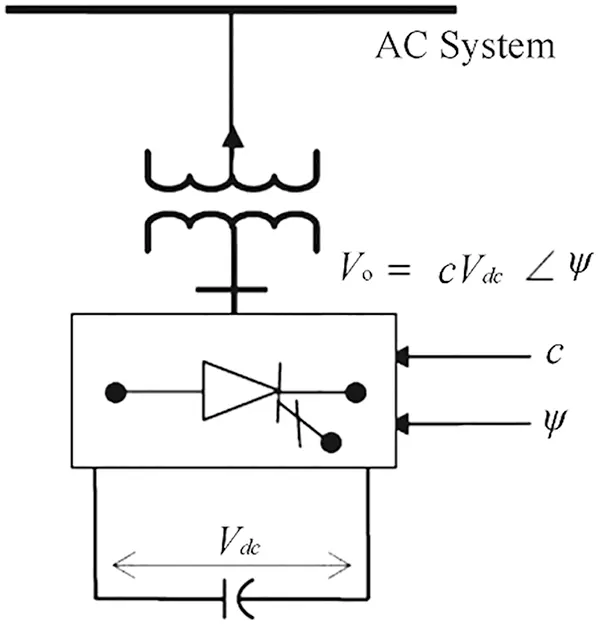Figure 1: D-STATCOM configuration 

In the D-STATCOM, a VSC generates a controllable AC voltage behind the leakage reactance of the transformer, and the voltage difference between the two reactance sides leads to the exchange of active and reactive power between the D-STATCOM and transmission grid. The output AC voltage of the VSC is controlled such that it is adequate to distribute the required reactive current. For each AC bus voltage, the voltage of the DC capacitor is automatically adjusted to function as the voltage source of the VSC. Hence, the D-STATCOM not only controls the voltage but also improves system damping. The D-STATCOM can be controlled by the voltage amplitude and phase angle control. Inverters in conventional D-STATCOM compensators are switched by a pulse in each period, and the transformer is used to minimize the harmonics. The control subsystem is designed using equipment that could continuously and rapidly control reactive power. The output voltage of the control subsystem consists of pulses very similar to the sinusoidal voltage. Active and reactive powers after the installation of the D-STATCOM are calculated as:

Pj=VjVeqXeqsin(αeqαj)(1)

Qj=VjVeqXeqcos(αeqαj)V2Xeq(2)

where

1Xeq=i=1n1Xi(3)

Veq=Xeq(A)2+(B)2(4)

αeq=tan1(AB)(5)

Also, A and B are given by:

A=i=1nViXisinαi(6)

B=i=1nViXicosαi(7)

As a result,

sin(αeqαj)=PjXeqVjVeq(8)

cos(αeqαj)=QjXeq+Vj2VjVeq(9)

The interaction between the AC voltage and the inverter-generated voltage adjusts and controls reactive power. The output power of the D-STATCOM is zero when the AC voltage and inverter-generated voltage are synchronous. However, when its output voltage is lower than the AC voltage, the D-STATCOM generates a voltage-proportional current that lags the voltage by 90 degrees, functioning as an inductive load. In such a case, reactive power is proportionate to the voltage magnitude. On the other hand, when the D-STATCOM voltage exceeds the system voltage, a leading current is generated that leads the voltage by 90 degrees, and the D-STATCOM functions as a capacitive load. In this case, reactive power is equivalent to the line voltage magnitude. The applied reactive power corresponds to the voltage. Thus, reactive power is controlled by controlling the voltage magnitude of the DC capacitor. The lagging or leading of the D-STATCOM voltage charges or discharges the capacitor in the DC part. As a result, the DC voltage changes, and the D-STATCOM displays the required indexes.

The D-STATCOM provides higher damping than the SVC in terms of dynamic stability improvement in the power system. It can exchange active power at a larger rate. The D-STATCOM is a fundamental FACTS device that can be used for voltage adjustment and dynamic voltage control in light of its excellent steady-state performance. It also can control the voltage amplitude at a small level and control the phase angle in a very short time. Thus, the D-STATCOM is properly capable of improving system fluctuations.

The control circuit plays a key role in the functionality of a compensator. An unsuitable controller design may adversely affect the compensator. Thus, it is required to employ an efficient control circuit in the D-STATCOM. Fig. 2 depicts the proposed control system for the D-STATCOM .Figure 2: Proposed control system

The voltage and current of the bus in which the D-STATCOM is installed are the inputs of the proposed control system. After passing the low-pass filters and discretization to generate voltage adjustment signals, the DC voltage adjustment is sent. Fig. 3 shows the block diagram of the voltage adjustment circuits.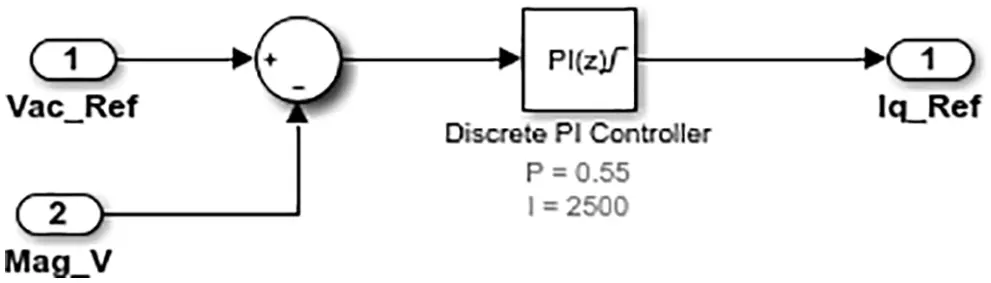Figure 3: Block diagram of voltage adjustment

Fig. 4 shows the block diagram of DC voltage control.Figure 4: Block diagram of DC voltage control

PI controllers were employed in both the voltage control and DC voltage control circuits to minimize the permanent fault of the compensator and enable the D-STATCOM to set the bus voltage to 1 pu. The coefficients of these two controllers were set by trials and errors–i.e., the coefficients were changed under different operating conditions to find the optimal values. Finally, the output signals of these two controllers are sent to generate the reference currents. Here, currents Id and Iq along with the reference Id and Iq generated by the voltage control and DC voltage control circuits are converted into the voltage magnitudes in Park’s domain. Then, the waveforms of voltages Vd and Vq can be used to generate signals to control the VSCs in the D-STATCOM.

4  DVR

Electronic devices (e.g., computers) sensitive to power quality are increasingly used in the world. Hence, it is necessary to develop equipment to improve power quality for consumers. In this respect, customer power devices were proposed to improve power quality in the 1980s. A DVR is a custom power compensator employed to compensate for the reduced/increased voltage.

Voltage sags are a common power quality issue that occurs in distribution systems. They refer to the instantaneous reduction in the root-mean-square (RMS) voltage by 0.9–1.1 pu. Voltage sags are assumed to be the most important power quality issue that typically arises from faults in the power system or the starting of large induction motors. They can interrupt or damage the function of electronic equipment that is sensitive to voltage variations in the power system. A voltage sag on the load side damages the equipment of clients. DVRs are utilized as a solution to voltage sags. In fact, a DVR is employed as a series device to inject voltage for sensitive loads when voltage variations occur. This is the main function of a DVR. Destructive fault currents are another problem of power systems. Fault current limiters are employed to avoid destructive fault effects. Fig. 5 illustrates the structure of a DVR .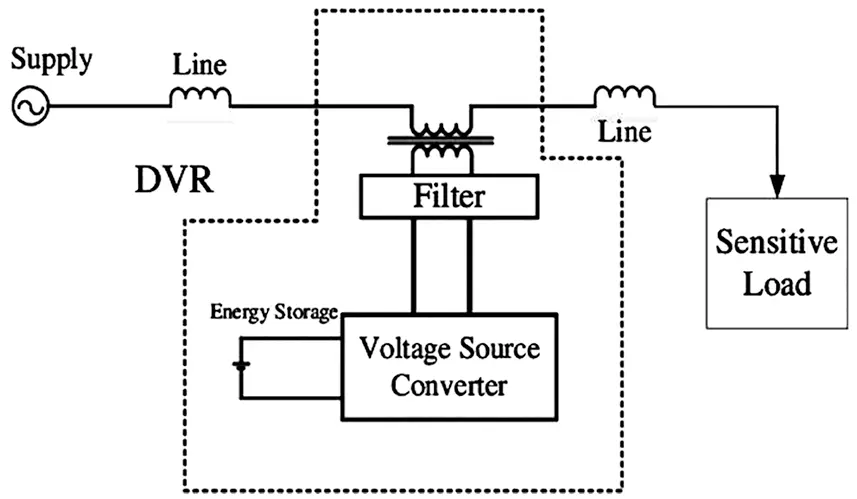Figure 5: Schematic of a DVR in a power system 

4.1 Description of DVR Components

A DVR consists of four major components, including:

VSC: A VSC is a power device that can generate a voltage at a given amplitude, phase, and frequency. VSCs mostly consist of IGBT and GTO switches and inject a controlled dynamic series three-phase voltage to the grid by a coupling transformer to stabilize the sensitive load voltage.

Coupling transformer: Three single-phase transformers are used to connect the VSC output to the distribution grid in series. These transformers are primarily responsible for enhancing the injected voltage and electrically insulating the VSC from the grid.

Passive filter: A capacitor is used as the filter in the coupling transformer to eliminate switching-induced harmonics and keeping the harmonics injected into the grid at the standard level.

Control system: The control system is primarily responsible for providing a suitable switching strategy to keep the voltage unchanged at the two ends of the sensitive load.

4.2 DVR Compensation of Voltage Reduction or Increase

Fig. 5 shows a DVR positioned in series within the circuit, where Vs is the source voltage, Xs is the source reactance, Vsc is the DVR-injected voltage, and XL is the effective reactance of the load segment. A voltage reduction on the source side would be detected by the control system, activating the switches so that the DVR generates the required voltage and compensates for the voltage reduction. Voltage reduction may have other reasons. In fact, a fault reduces V¯P. In normal conditions,

VL=VSjXSIL(10)

where V¯L is the voltage phasor (on the load side), and I¯L is the normal line current. The source voltage is modeled by V¯sag when a fault occurs in the source and reduces V¯p (i.e., a voltage sag). In the absence of series compensation:

VP=VL´=VsagjXSI´L(11)

where V¯L, V¯p, and I¯L are the load-side voltage, bus voltage, and load current under a voltage sag. To protect the load from such a sag, the DVR should inject V¯sc to the grid in series to restore the load voltage to the pre-fault level. As a result,

VL=VsagjXSIL+VSC(12)

It is required to obtain V¯sc. In fact, V¯sc injected by the DVR can be obtained at a given time by measuring V¯P in the normal conditions and measuring V¯P online under fault conditions. Then, instructions are sent by the control system to the DVR switches, injecting the required voltage to the grid. Voltage increase/reduction may be symmetric and asymmetric and occur with or without a voltage phase shift. To compensate for a voltage increase or reduction, it is required to calculate the voltage that needs to be generated by the DVR (i.e., the reference voltage). The reference voltage varies, depending on the control strategy. Figs. 6 to 8 represent the phasor diagrams of different control strategies.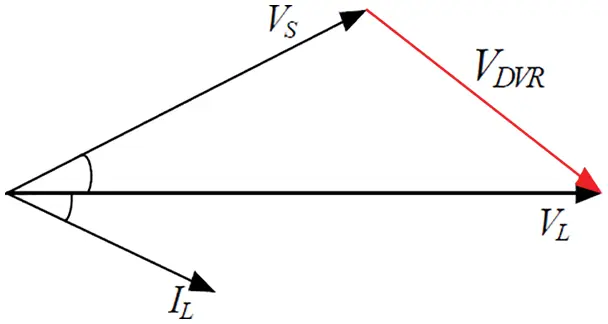Figure 6: Phasor diagram of pre-disturbance strategyFigure 7: Phasor diagram of the in-phase strategy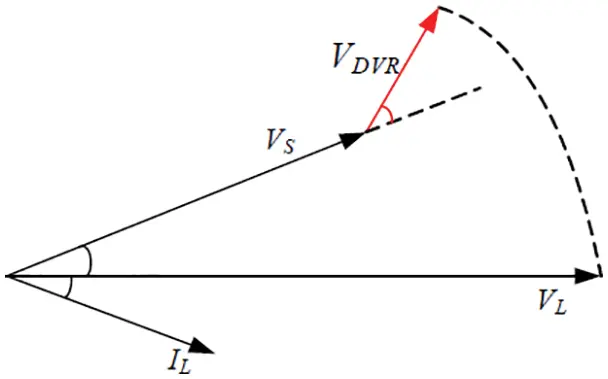Figure 8: Phasor diagram of the minimum-phase strategy 

Fig. 6 depicts the phasor diagram of the pre-disturbance strategy. As can be seen, the DVR implements compensation such that the compensated load voltage has the same magnitude and phase as the pre-disturbance voltage. This strategy is useful for loads that are sensitive to the voltage phase, such as thyristor-controlled loads. Fig. 7 illustrates the phasor diagram of the in-phase strategy, in which DVR injects a voltage with the same phase as the post-disturbance voltage. In both pre-disturbance and in-phase strategies, the DVR injects active power to the grid. The minimum-phase strategy is adopted to reduce the injected active power. In this strategy, the DVR injects a voltage with a 90-degree phase difference from the line current, reducing the active power injection to zero.

These three strategies exploit PLL to obtain the voltage phase. Fault currents are important in power systems. Such currents in power systems can damage transformers and other expensive equipment. Therefore, relays and circuit breakers are employed to avoid such destructive currents. However, relays and circuit breakers typically have delays. Moreover, energy interruption in a part of a power system would impose many financial losses. Thus, fault current limiters have been discussed in recent years .

5  Results and Discussion

This section provides the numerical MATLAB Simulink results. Figs. 9 and 10 depict the single-line model of the system under study and the simulated model of the power system, respectively. The system under study is a standard IEEE 13-bus power system functioning as an unbalanced distribution system in the presence of wind power generation in which single- and three-phases are fed.Figure 9: Single-line model of the power systemFigure 10: Simulated model of the power system

The power system has a wind farm with 1 MW generators connected to the grid through a 4 MVA transformer and a 600-m line, as shown in Fig. 11.Figure 11: Wind farm

The wind farm generator was an induction generator. The turbine had a cut-out speed of 16 m/s and a cut-in speed of 4 m/s. The rated wind speed was 10 m/s. Fig. 12 represents the power factor of the wind turbine at different wind speeds.Figure 12: Power factor of the wind turbine vs. wind speed

Compensation circuits significantly contribute to voltage stability improvement in power systems. As mentioned, the present study separately employed a synchronous static compensator (i.e., D-STATCOM) and a DVR to improve the voltage stability of the power system in the connection line between the wind farm and power grid .

Fig. 13 depicts the connection of the D-STATCOM in the power system. Synchronous static compensators are used in parallel in the wind farm-grid connection line. The D-STATCOM injects reactive power when a fault occurs in order to stabilize the voltage.Figure 13: D-STATCOM connection to the power system

Fig. 14 illustrates the DVR in the distribution system. The DVR is positioned in the connection line between the wind turbine and power grid in series. It avoids large voltage variations (and voltage instability) by injecting compensating voltage.Figure 14: DVR connection to the power system

Fig. 15 indicates the block diagram of the simulated DVR.Figure 15: Simulated DVR model

A DVR compensates for the voltage to bring the grid voltage back at the acceptable level when a fault occurs in the system. The DVR and D-STATCOM compensators were evaluated in two scenarios. The first scenario evaluated the performance of the compensators in improving system unbalance, while the second one evaluated their performance under short-term overload conditions.

5.1 Scenario 1

The performance of the proposed DVR and D-STATCOM was evaluated under a 2 MVA 1-phase load applied from 1.5  to 2.5 s in one of the phases (system unbalance). It should be noted that the system was already unbalanced in normal conditions due to single-phase loads, and the added single-phase load increased the unbalance. Also, fixed wind speeds of 8 and 12 m/s were assumed to incorporate wind power. Fig. 16 depicts the system voltage in the absence of compensators.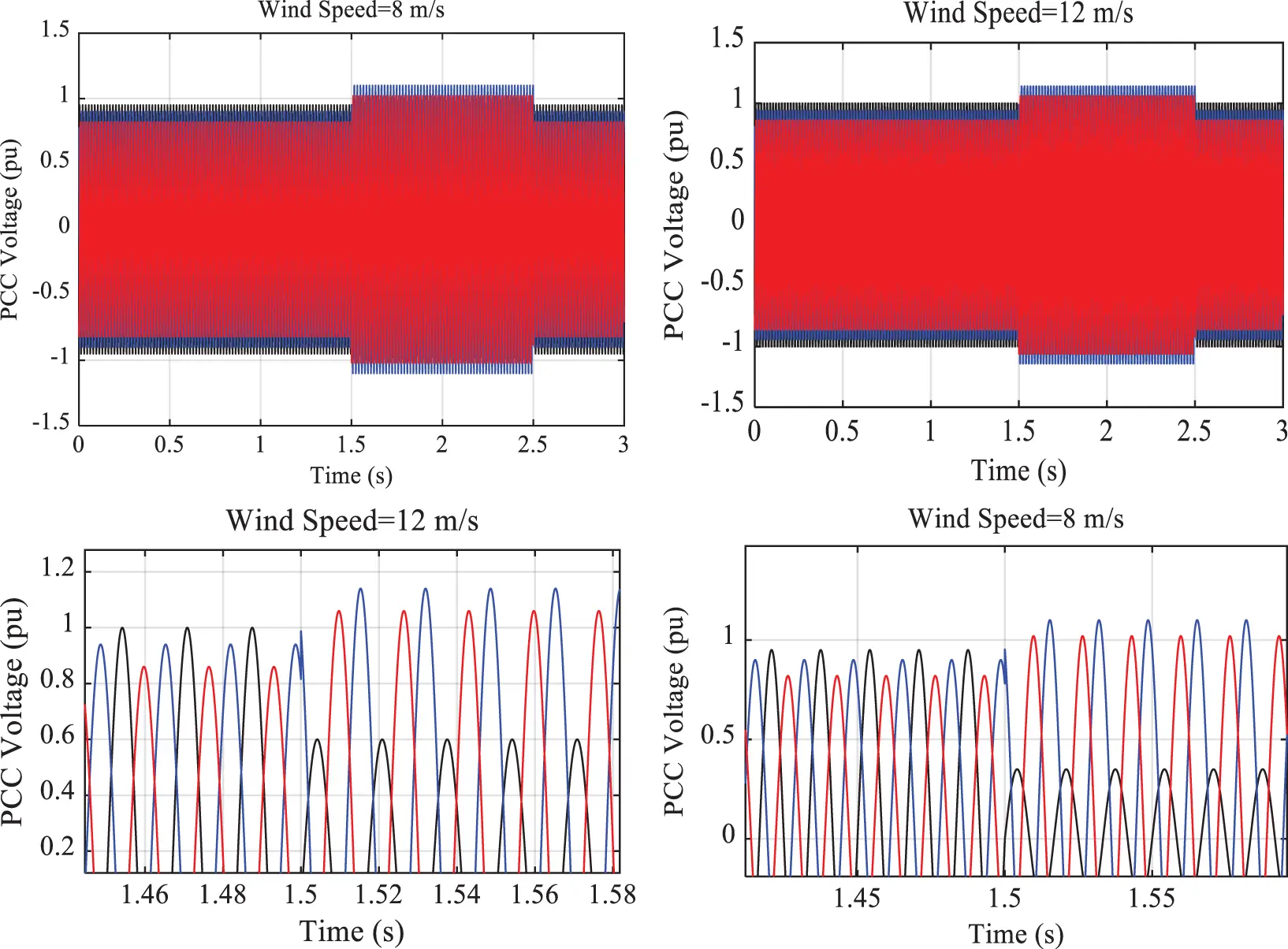Figure 16: PCC bus voltage in the absence of compensators

The PCC bus voltage was below 1 pu in all phases under normal conditions at a wind speed of 8 m/s. However, at a wind speed of 12 m/s, the PCC bus voltage sag was lower due to the larger power generation of the wind farm, and the phase voltage amplitude was closer to 1 pu. The application of a single-phase load to the grid increased the unbalance. As a result, the voltage amplitude of the corresponding phase became nearly 0.4 pu at a wind speed of 8 m/s and approximately 0.6 pu at a wind speed of 12 m/s. To more accurately compare the results, the voltage deviation index (VDI) was calculated for the pre-fault and fault conditions as:

%VDI=(VnVminVn)×100(13)

where Vn is the rated voltage of the grid, while Vmin is the minimum voltage amplitude among the three phases. The VDI of the uncompensated system was calculated to be 18% and 15% at the wind speeds of 8 and 12 m/s, respectively. Also, the VDI was obtained to be 65% and 40% under fault conditions at the wind speeds of 8 and 12 m/s, respectively. Fig. 17 plots the PCC bus voltage when the D-STATCOM was employed.Figure 17: PCC bus voltage in the presence of the D-STATCOM under Scenario 1

It was found that the D-STATCOM reduced the pre-fault voltage unbalance, and the VDI reduced below 7% and 4% at the wind speeds of 8 and 12 m/s, respectively. Under fault conditions, on the other hand, the VDI was calculated to be 15% and 11% at the wind speeds of 15% and 11%, respectively. Fig. 18 depicts the D-STATCOM voltage injection.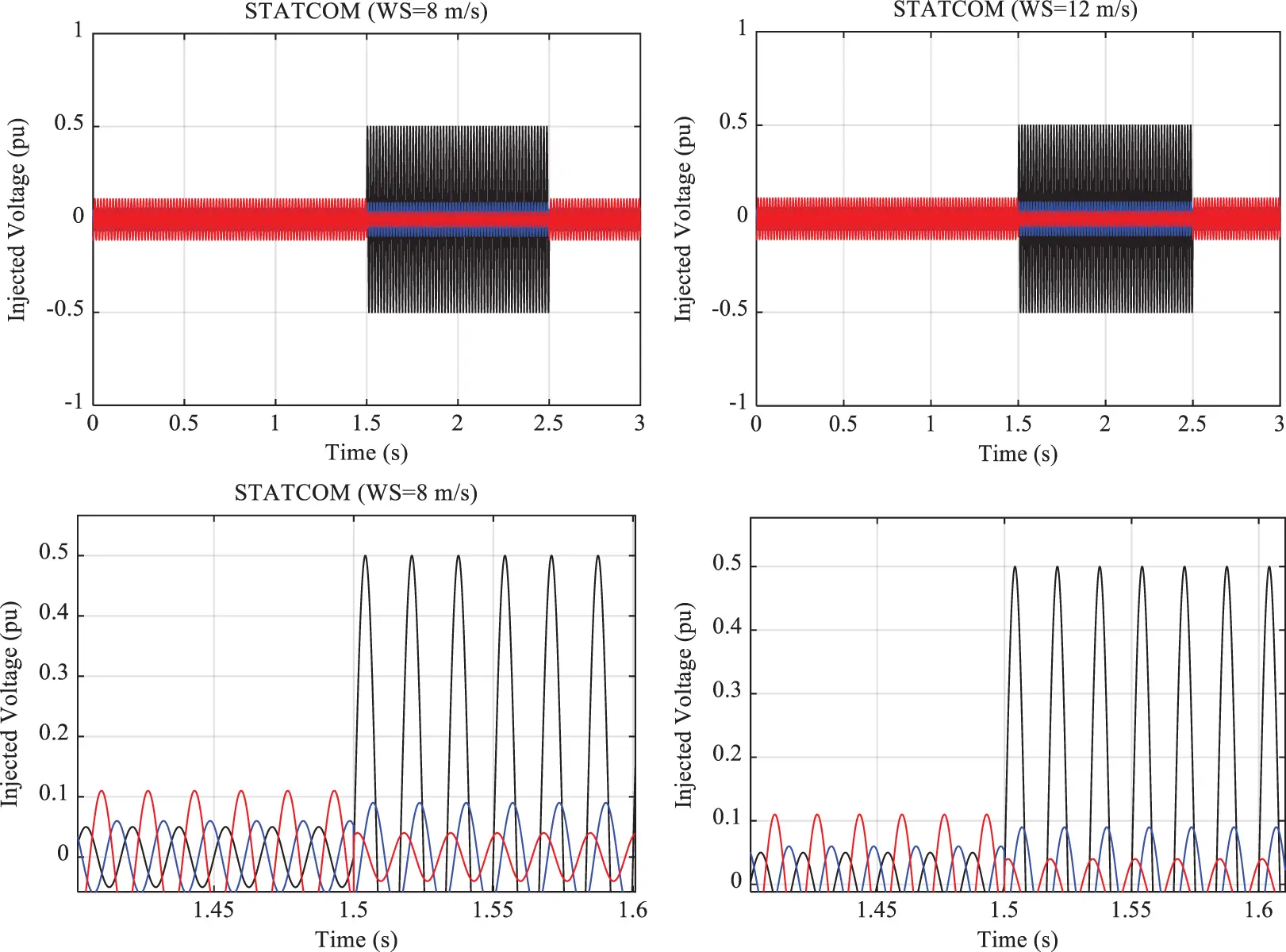Figure 18: D-STATCOM voltage injection under Scenario 1

As can be seen, D-STATCOM voltage injection was insignificant under normal conditions. In fact, the D-STATCOM only reduced the system unbalance and improved power quality. Once the single-phase load was applied to the system, the voltage injection increased to prevent the grid voltage amplitude from exceeding the permissible level. The D-STATCOM showed higher performance at a wind speed of 12 m/s than at 8 m/s since the wind farm generated the rated power at 12 m/s, while its power generation at 8 m/s was lower than the rated value. Fig. 19 shows the PCC bus voltage in the presence of the DVR.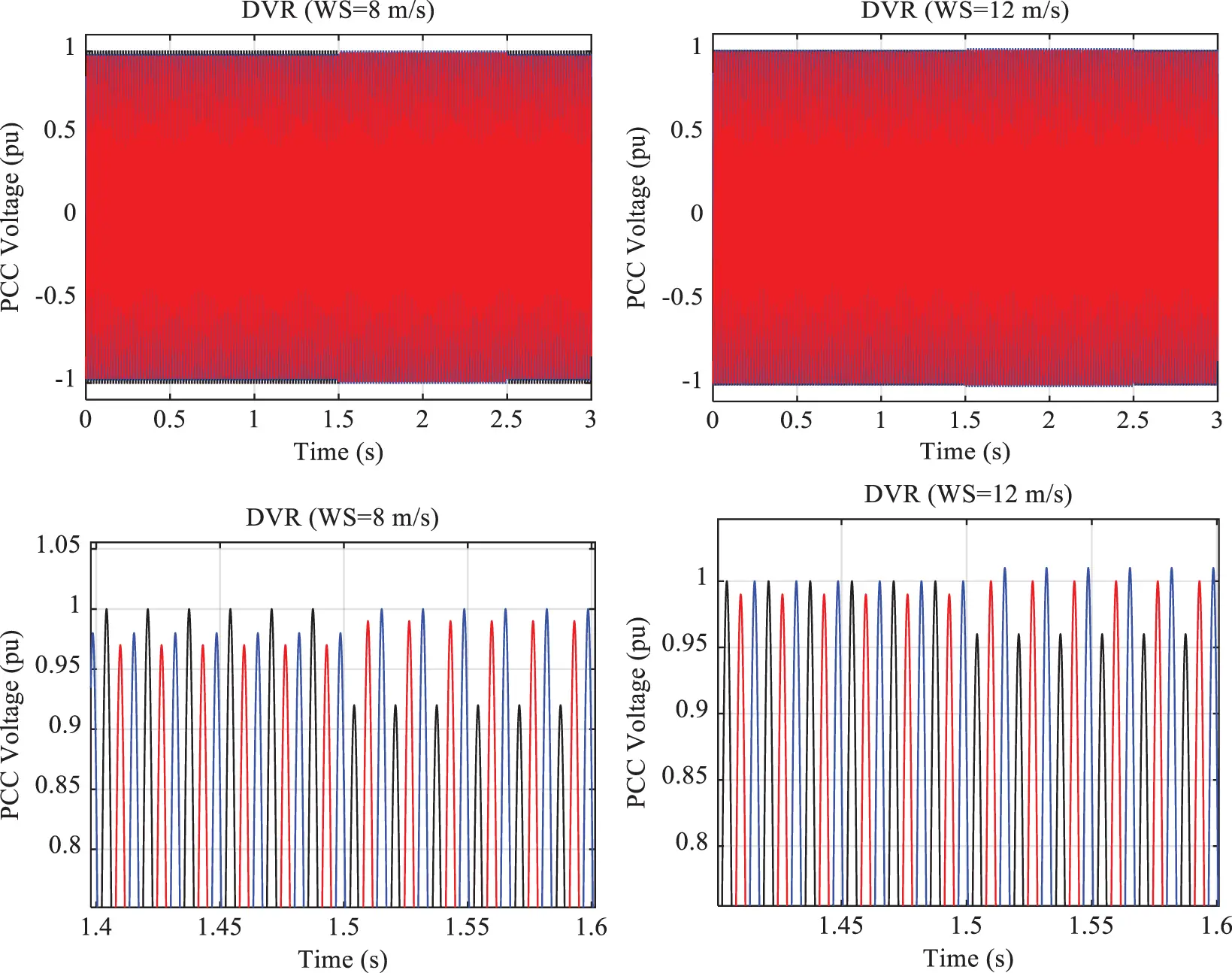Figure 19: PCC bus voltage in the presence of the DVR

As can be seen, the DVR improved the voltage amplitude, and the VDI declined below 4% under normal conditions. The added single-phase load changed the VDI to nearly 8% at the wind speed of 8 m/s and 4% at the wind speed of 12 m/s. Fig. 20 represents the DVR voltage injection.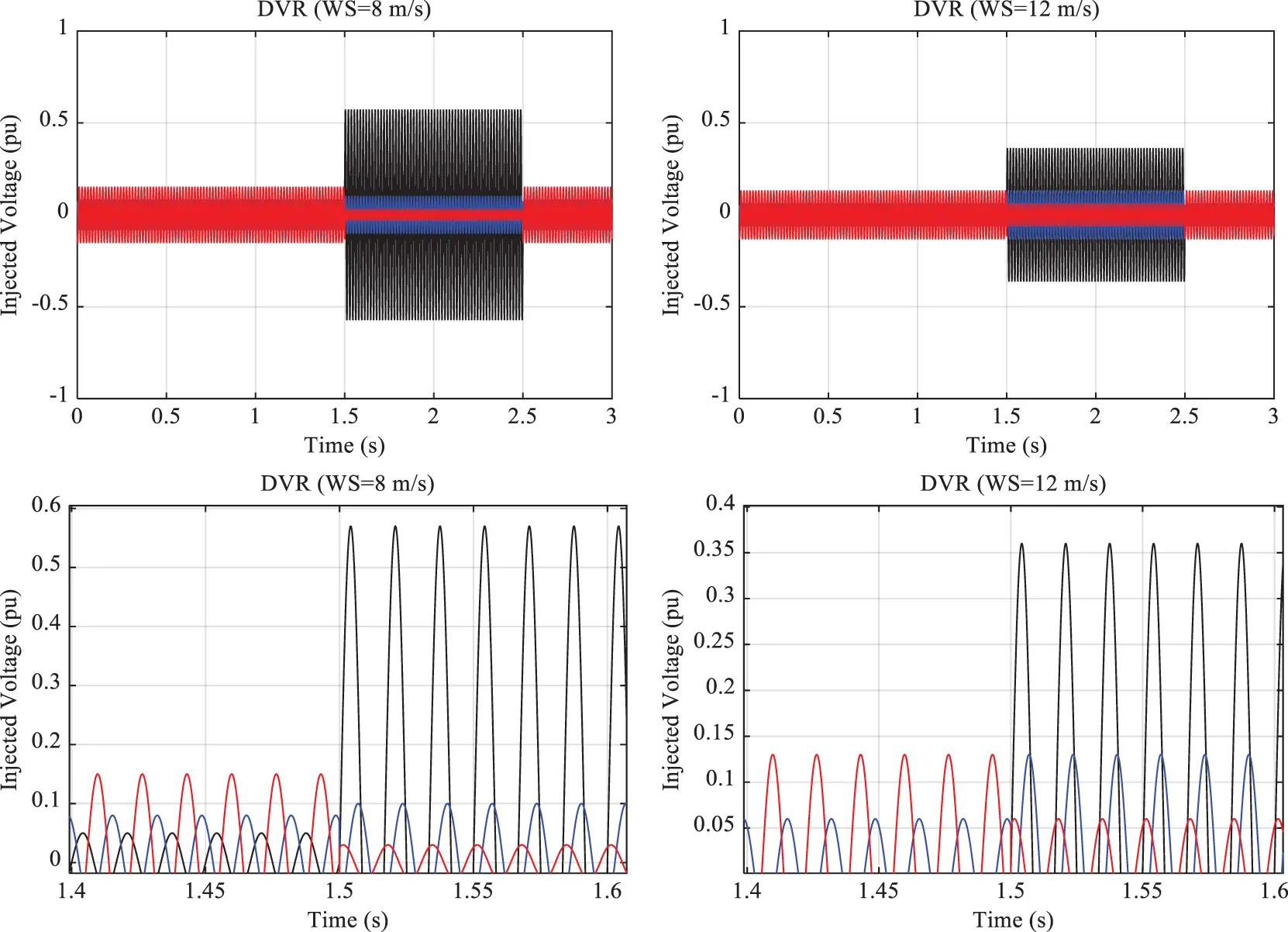Figure 20: DVR voltage injection

As can be seen in Fig. 20, the DVR injected insignificant voltage under normal conditions. However, the DVR voltage injection increased under fault conditions to compensate for the voltage reduction caused by the fault (i.e., the single-phase load). Furthermore, due to the system unbalance, voltage injection differed in different phases. Fig. 21 shows the VDI bar charts under Scenario 1.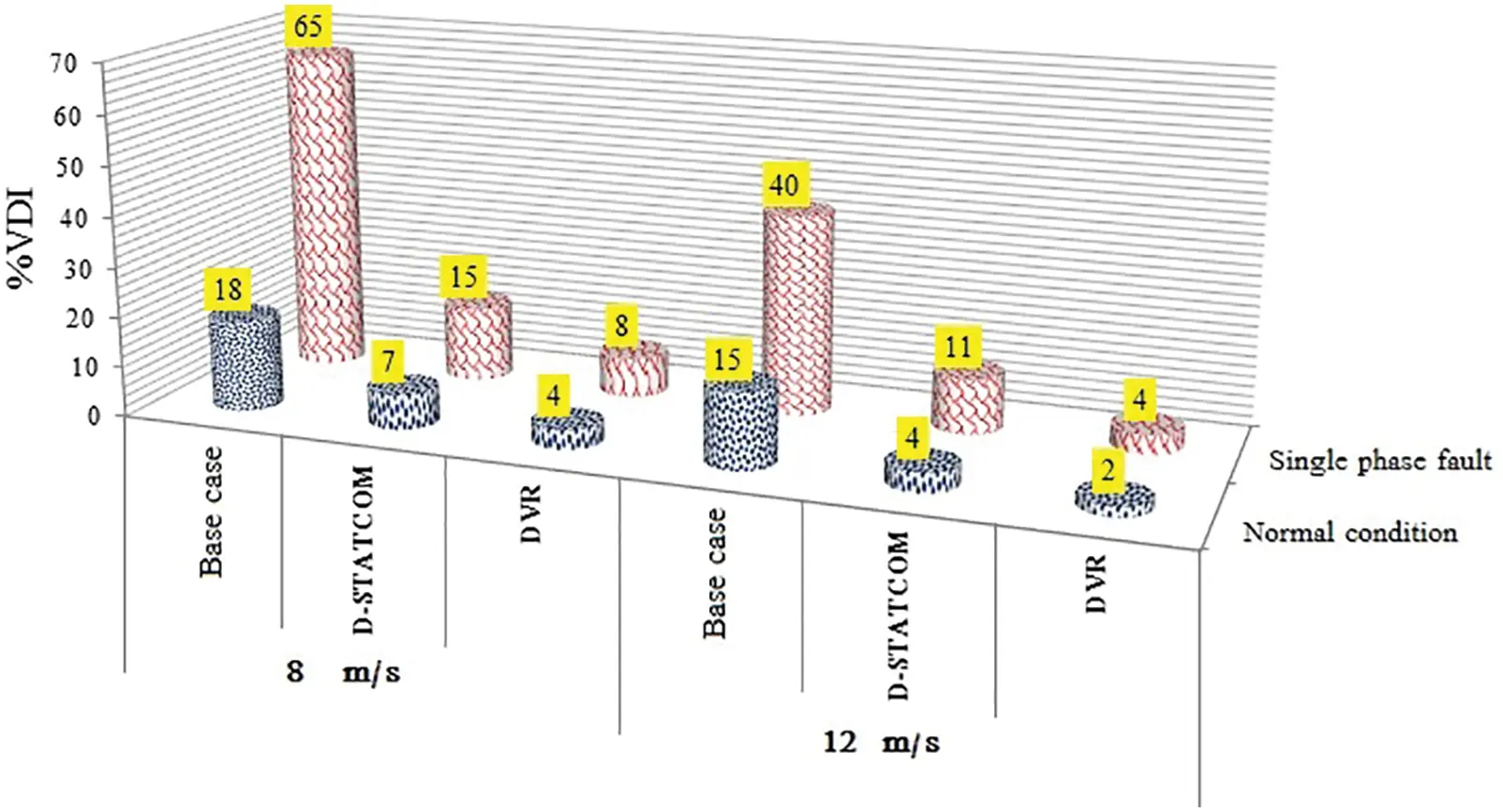Figure 21: Simulation results under Scenario 1

According to Fig. 21, the VDI was large in the absence of a compensator. This suggests an undesirable grid voltage. The VDI increased to 65% under the single-phase load at a wind speed of 8 m/s. The compensators, however, improved the voltage, significantly reducing the VDI. The DVR and D-STATCOM showed almost the same behavior under normal conditions. However, the DVR outperformed the D-STATCOM under fault conditions, leading to smaller VDI values at both wind speeds of 8 and 12 m/s. Under normal conditions, the DVR reduced the VDI below 4%. This demonstrates the high performance of the DVR in balancing the PCC bus voltage.

5.2 Scenario 2

Compensators should perform properly under any system loads and reduce the VDI to avoid voltage instability. Scenario 2 measured the performance of the compensators under a balanced three-phase load. Similar to Scenario 1, the system was loaded at 2.5 s and unloaded at 3.5 s in Scenario 2. Likewise, wind speeds of 8 and 12 m/s were applied to Scenario 2. Fig. 22 plots the PCC bus voltage under Scenario 2.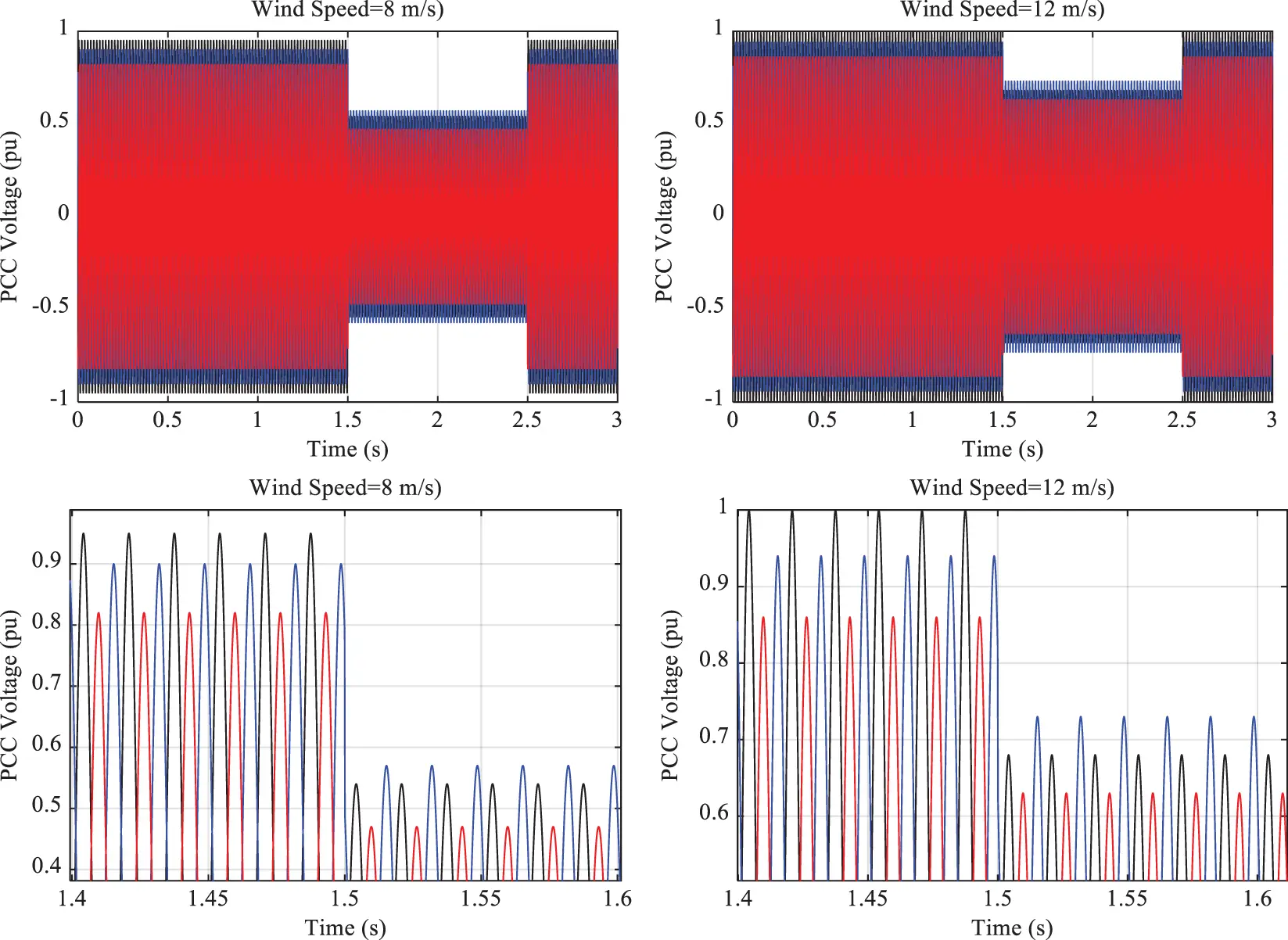Figure 22: PCC bus voltage in the absence of compensators under Scenario 2

As can be seen in Fig. 22, the voltage amplitudes of all the phases declined immediately after the three-phase load was applied. This suggests poor voltage stability in the grid. Also, the voltage amplitude of one of the phases reduced to nearly 0.46 and 0.63 pu at the wind speeds of 8 and 12 m/s, respectively. Thus, it is required to employ compensators in this feeder. Fig. 23 shows the system voltage amplitude in the presence of the D-STATCOM under Scenario 2. It was found that the VDI in normal conditions under Scenario 2 was similar to that under Scenario 1. Under fault conditions, however, the VDI was calculated to be approximately 12% and 7% at the wind speeds of 8 and 12 m/s, respectively.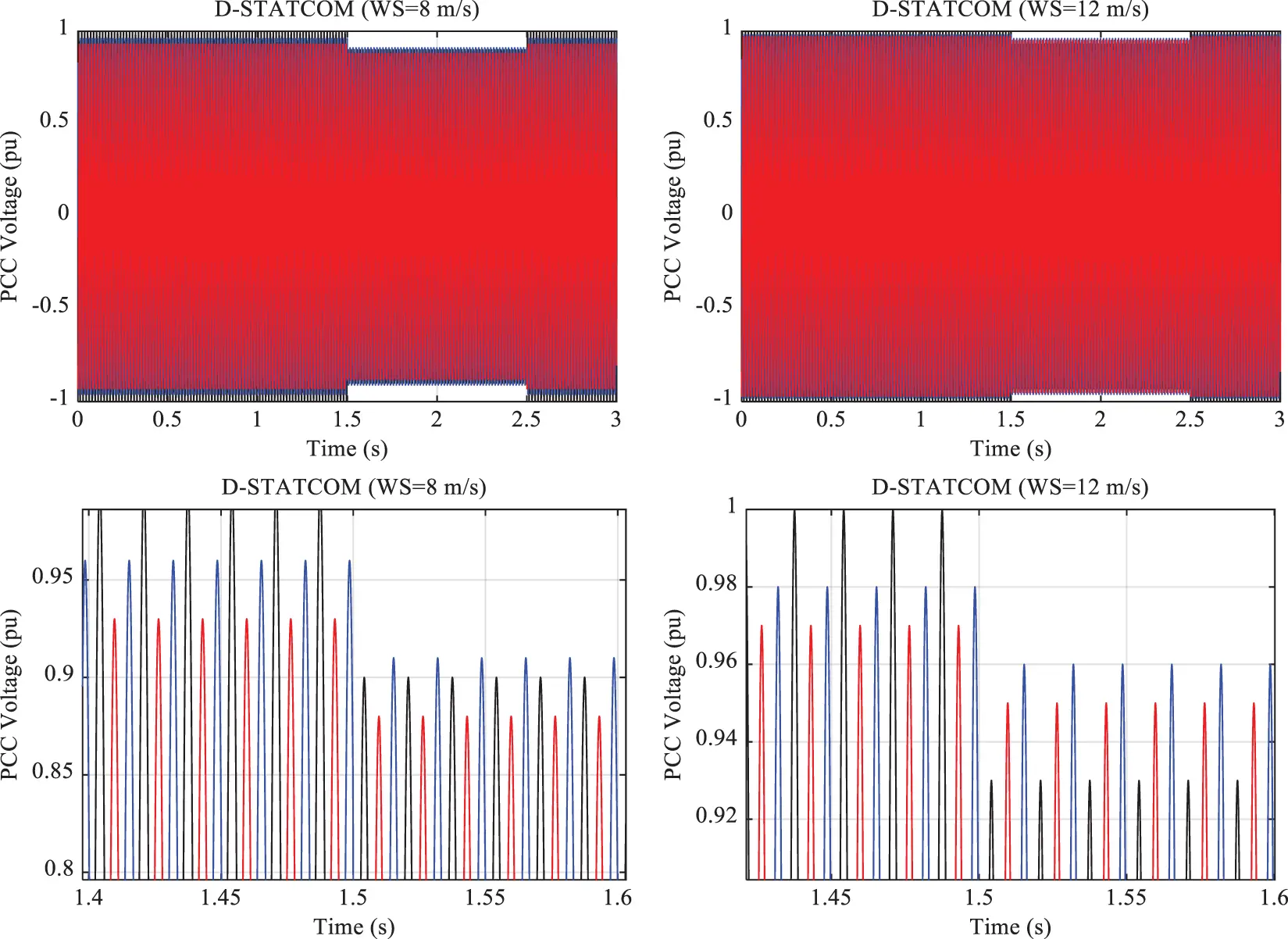Figure 23: PCC bus voltage in the presence of the D-STATCOM under Scenario 2

Fig. 24 depicts the D-STATCOM voltage injection. As can be seen, D-STATCOM implemented a larger voltage injection at a wind speed of 12 m/s than at 8 m/s. Also, the voltage injection was found to be insignificant enough to experience voltage balance in the phases under normal conditions. Fig. 25 plots the PCC bus voltage in the presence of the DVR vs. wind farm power.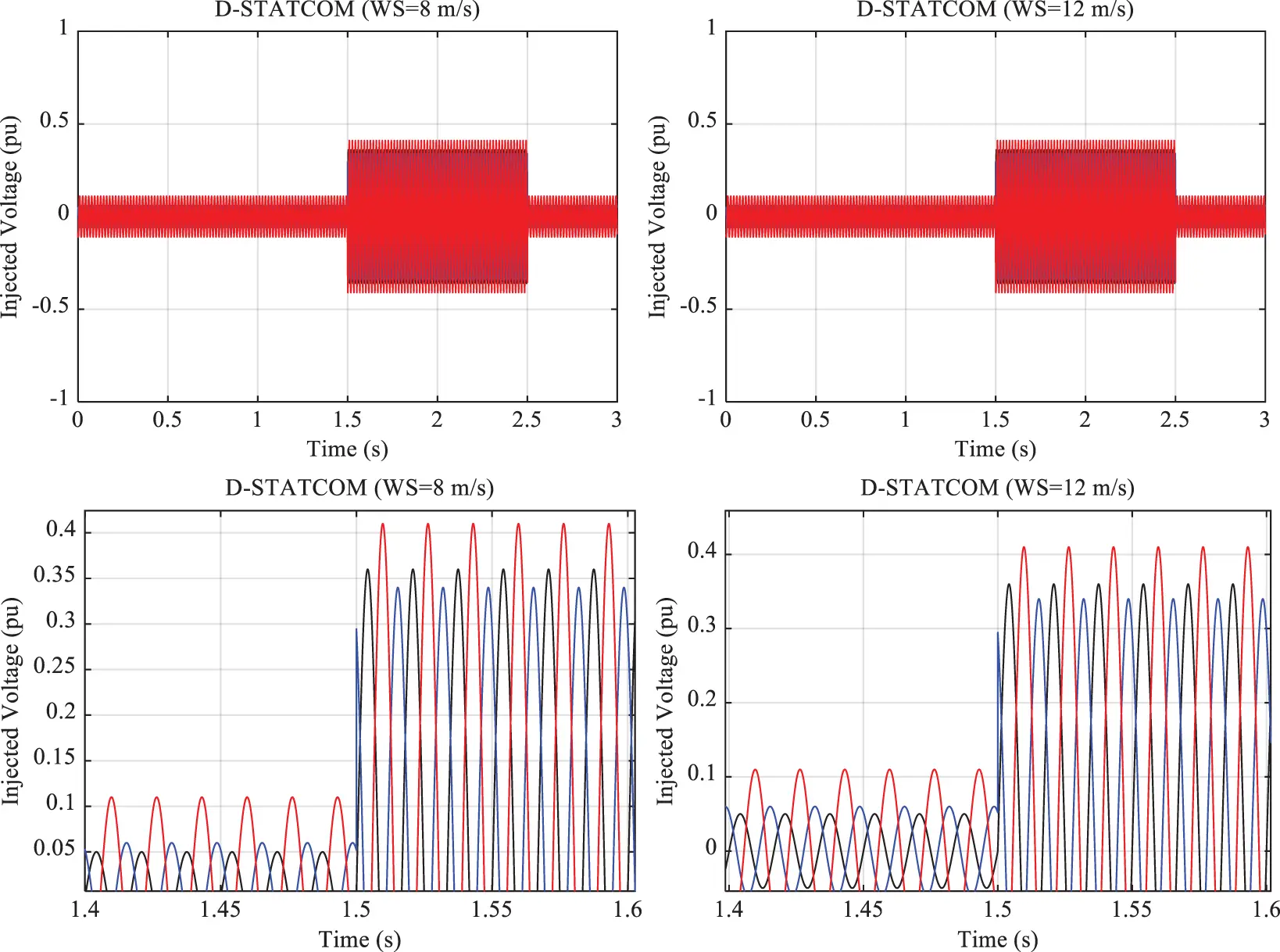Figure 24: D-STATCOM voltage injection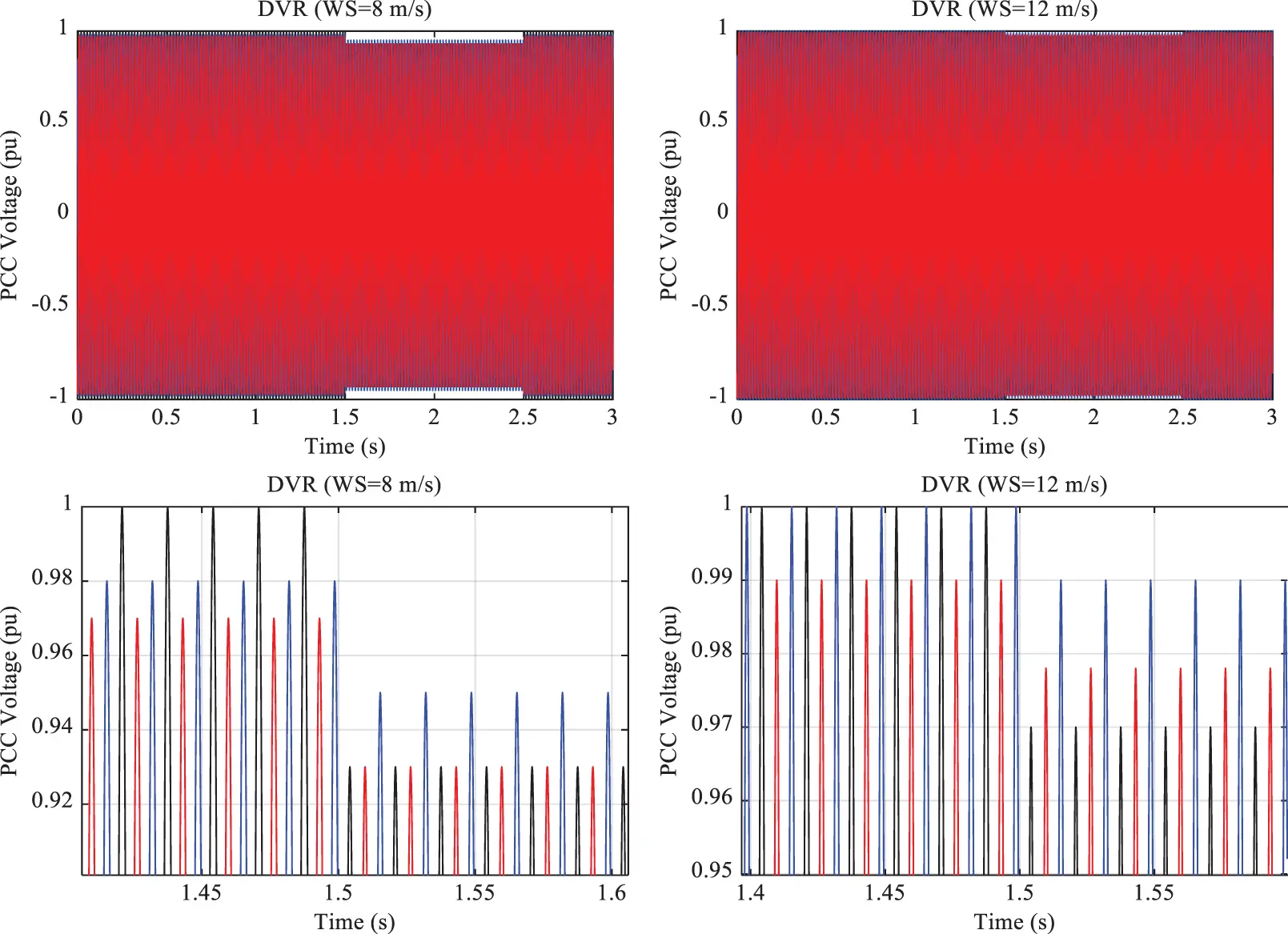Figure 25: PCC bus voltage in the presence of the DVR under Scenario 2

According to Fig. 25, it can be said that the system voltage was stable in the presence of the DVR; the voltage amplitude remained in the permissible range after the three-phase load was applied. Under the DVR and fault conditions, the VDI was obtained to be 7% and 3% at the wind speeds of 8 and 12 m/s, respectively. Fig. 26 shows the DVR voltage injection under Scenario 2.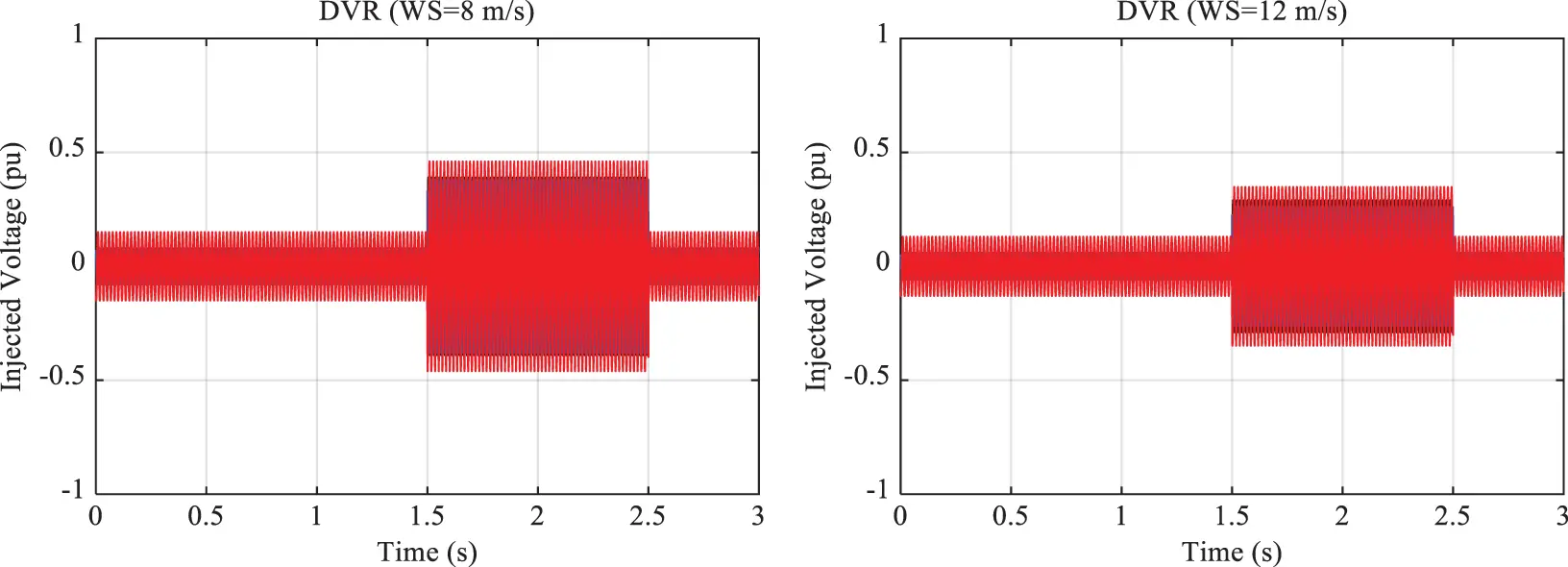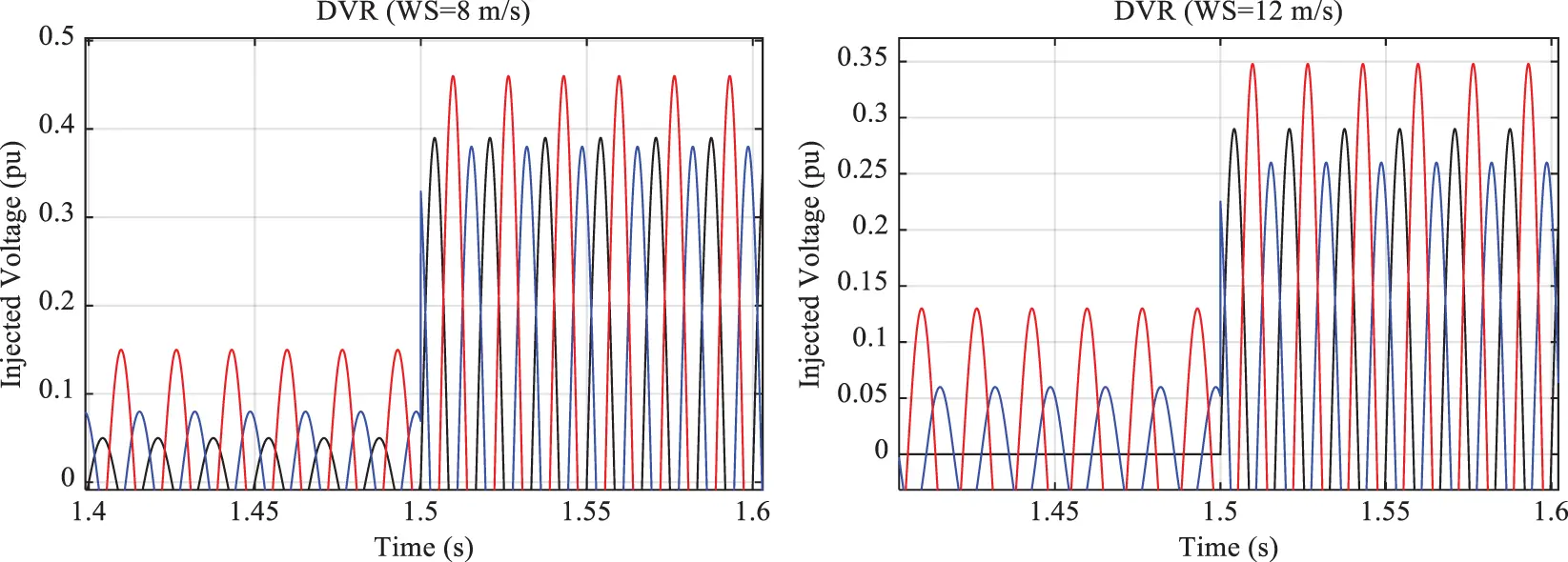Figure 26: DVR voltage injection under Scenario 2

Fig. 27 depicts the VDI under Scenario 2. As can be seen, the VDI in Scenario 2 was similar to that in Scenario 3 under normal conditions. However, the VDI changed when the three-phase load was applied to the system.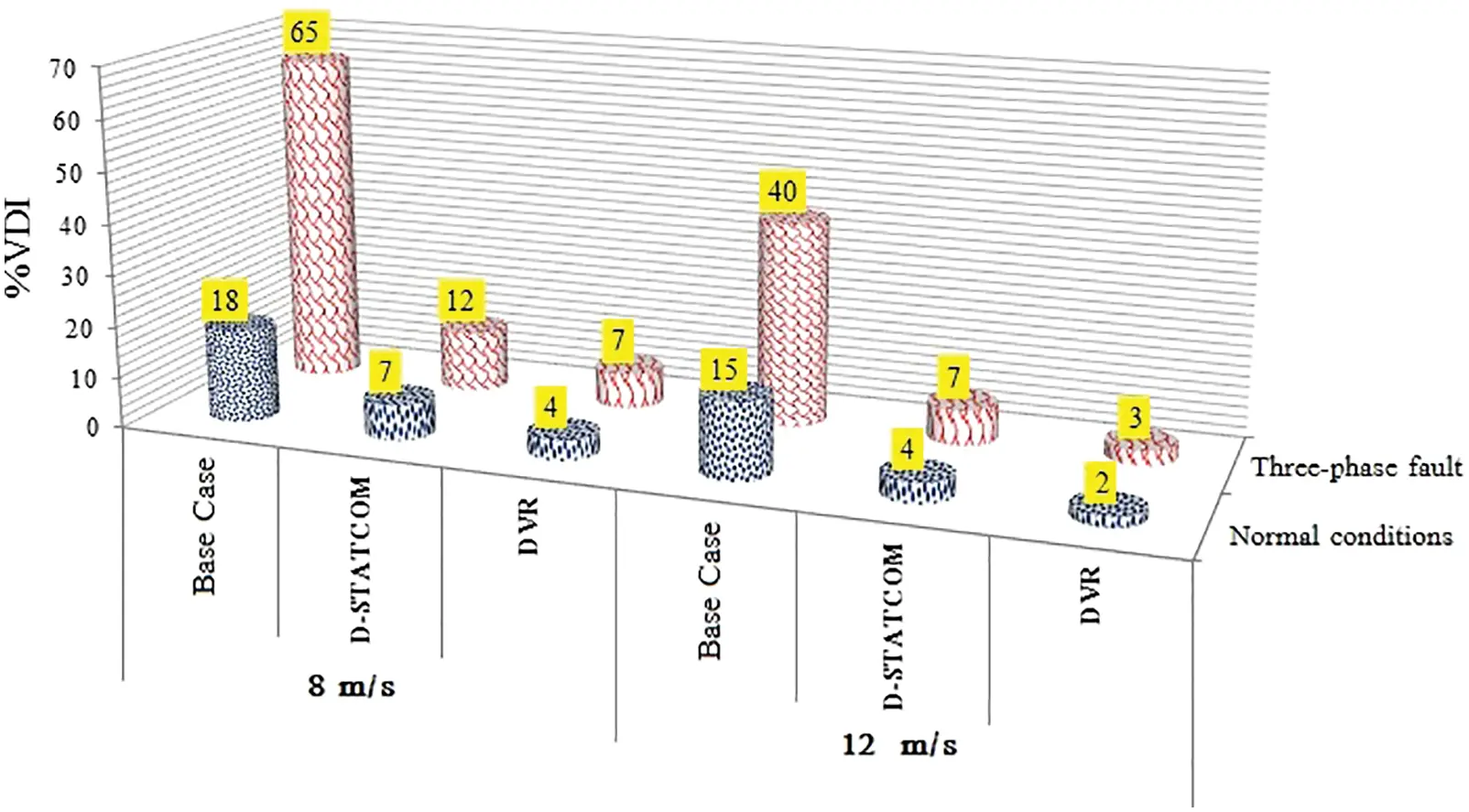Figure 27: Simulation results under Scenario 2

As with Scenario 1, the DVR outperformed the D-STATCOM under Scenario 2. The VDI was obtained to be smaller for the DVR than for D-STATCOM under fault conditions.

6  Conclusion

The present study evaluated the performance of the D-STATCOM and DVR compensators in a distribution system under unbalanced loads. The power system was simulated in MATLAB R2017b (Version 9.3). The single- and three-phase loads were applied under two scenarios to measure the performance of the compensators. The results demonstrated that the voltage of the grid would be unstable in the absence of compensators, particularly under fault conditions. In such a case, the voltage amplitude of the grid would dramatically decline. Thus, it was necessary to utilize compensators. Moreover, it was found that the two compensators had almost the same performance under normal conditions. Under fault conditions (single- and three-phase faults), however, the DVR outperformed the D-STATCOM and prevented dramatic voltage amplitude variations.

Funding Statement: This work was supported in part by an International Research Partnership “Electrical Engineering–Thai French Research Center (EE-TFRC)” under the project framework of the Lorraine Université d’Excellence (LUE) in cooperation between Université de Lorraine and King Mongkut’s University of Technology North Bangkok and in part by the National Research Council of Thailand (NRCT) under Senior Research Scholar Program under Grant No. N42A640328, and in part by National Science, Research and Innovation Fund (NSRF) under King Mongkut’s University of Technology North Bangkok under Grant No. KMUTNB-FF-65-20.

Conflicts of Interest: The authors declare that they have no conflicts of interest to report regarding the present study.

## References

1. M. A. El-Gammal, A. Y. Abou-Ghazala and T. I. El-Shennawy, “Dynamic voltage restorer (DVR) for voltage sag mitigation,” International Journal on Electrical Engineering and Informatics, vol. 3, pp. 1–11, 2015.
2. N. W. A. Lidula and A. D. Rajapakse, “Voltage balancing and synchronization of microgrids with highly unbalanced loads,” Renewable and Sustainable Energy Reviews, vol. 31, pp. 907–920, 2014.
3. N. Rezaei, M. Kalantar, H. A. Shayanfar, Y. Alipouri and A. Safari, “Optimal IPFC signal selection and damping controller design using a novel current injection model in a multi-machine power system,” International Journal of Electrical Power & Energy Systems, vol. 44, no. 1, pp. 641–470, 201
4. L. Ye, Y. Zhao, C. Zeng and C. Zhang, “Short-term wind power prediction based on spatial model,” Renewable Energy, vol. 101, pp. 1067–1074, 2017.
5. N. Amutha and B. Kalyan Kumar, “Improving fault ride-through capability of wind generation system using DVR,” International Journal of Electrical Power & Energy Systems, vol. 46, pp. 326–333, 2013.
6. B. Ferdi, C. Benachaiba, S. Dib and R. Dehini, “Adaptive PI control of dynamic voltage restorer using fuzzy logic,” Journal of Electrical Engineering: Theory and Application, vol. 1, no. 3, pp. 165–173, 2010.
7. M. Ahmadi Kamarposhti, H. Shokouhandeh, M. Alipur, I. Colak, H. Zare et al., “Optimal designing of fuzzy-PID controller in the load-frequency control loop of hydro-thermal power system connected to wind farm by HVDC lines,” IEEE Access, vol. 10, pp. 63812–63822, 2022.
8. M. A. Kamarposhti, H. Shokouhandeh, I. Colak, S. S. Band and K. Eguchi, “Optimal location of FACTS devices in order to simultaneously improving transmission losses and stability margin using artificial bee colony algorithm,” IEEE Access, vol. 9, pp. 125920–125929, 2021.
9. H. Abdollahzadeh, M. Jazaeri and A. Tavighi, “Design a new fast-converged estimation approach for dynamic voltage restorer (DVR) to compensate voltage sags in waveform distortion conditions,” International Journal of Electrical Power and Energy Systems, vol. 54, pp. 598–609, 2014.
10. N. Saudin, “Voltage sags mitigation techniques analysis,” Ph.D. Dissertation, Universiti Teknologi Malaysia, Malaysia, 2007.
11. R. Omar, N. A. Rahim and M. Sulaiman, “Dynamic voltage restorer application for power quality improvement in electrical distribution system: An overview,” Australian Journal of Basic and Applied Sciences, vol. 5, no. 12, pp. 379–396, 20
12. C. O. Adika and L. Wang, “Autonomous appliance scheduling for household energy management,” IEEE Transactions on Smart Grid, vol. 5, no. 2, pp. 673–682, 2013.
13. X. Chen, T. Wei and S. Hu, “Uncertainty-aware household appliance scheduling considering dynamic electricity pricing in smart home,” IEEE Transactions on Smart Grid, vol. 4, no. 2, pp. 932–941, 20
14. M. Tarafdar, A. Shaker and F. Sohrabi, “Fuzzy-based controller for DVR in the presence of DG,” Procedia Computer Science, vol. 120, pp. 684–690, 2017.
15. R. Moslemi and H. A. Shayanfar, “Optimal location for series FACTS devices to transient stability constrained congestion management,” in 10th Int. Conf. on Environment and Electrical Engineering, Rome, Italy, pp. 1–4, 2011.
16. X. Guan, Z. Xu and Q. S. Jia, “Energy-efficient buildings facilitated by microgrid,” IEEE Transactions on Smart Grid, vol. 1, no. 3, pp. 243–252, 2010.
17. R. Boqiang and J. Chuanwen, “A review on the economic dispatch and risk management considering wind power in the power market,” Renewable and Sustainable Energy Reviews, vol. 13, no. 8, pp. 2169–2174, 2009.
18. P. Kundur, J. Paserba, V. Ajjarapu, G. Andersson, A. Bose et al., “Definition and classification of power system stability IEEE/CIGRE joint task force on stability terms and definitions,” IEEE Transactions on Power Systems, vol. 19, no. 3, pp. 1387–1401, 2004.
19. N. Mithulananthan and S. C. Srivastava, “Investigation of a voltage collapse incident in Sri Lankan power system network,” in Int. Conf. on Energy Management and Power Delivery (Cat. No. 98EX137), Singapore, vol. 1, pp. 47–53, 1998.
20. S. Panda, “Multi-objective PID controller tuning for a FACTS-based damping stabilizer using non-dominated sorting genetic algorithm-II,” International Journal of Electrical Power and Energy Systems, vol. 33, pp. 1296–1308, 2011.
21. M. Nabipour, M. Razaz, G. Seifossadat and S. Motazavi, “A novel adaptive fuzzy membership function tuning algorithm for robust control of a PV-based dynamic voltage restorer (DVR),” Engineering Applications of Artificial Intelligence, vol. 53, pp. 155–175, 2016.
22. S. Agalar and Y. Kaplan, “Power quality improvement using STS and DVR in wind energy system,” Renewable Energy, vol. 118, pp. 1031–1040, 2018.
23. L. Zhang, H. Zheng, G. Cai, Z. Zhang, X. Wang et al., “Power-frequency oscillation suppression algorithm for AC microgrid with multiple virtual synchronous generators based on fuzzy inference system,” IET Renewable Power Generation, vol. 16, no. 8, pp. 1589–1601, 2022.
24. L. Zhang, T. Gao, G. Cai and K. L. Hai, “Research on electric vehicle charging safety warning model based on back propagation neural network optimized by improved gray wolf algorithm,” Journal of Energy Storage, vol. 49, pp. 104092, 2022.
25. L. Zhang, H. Zhang and G. Cai, “The multi-class fault diagnosis of wind turbine bearing based on multi-source signal fusion and deep learning generative model,” IEEE Transactions on Instrumentation and Measurement, vol. 71, pp. 1–12, 2022.
26. D. Yu, Z. Ma and R. Wang, “Efficient smart grid load balancing via fog and cloud computing,” Mathematical Problems in Engineering, vol. 2022, pp. 1–11, 2022.
27. D. Yu, J. Wu, W. Wang and B. Gu, “Optimal performance of hybrid energy system in the presence of electrical and heat storage systems under uncertainties using stochastic p-robust optimization technique,” Sustainable Cities and Society, vol. 83, pp. 103935, 2022.
28. T. Cai, Y. Dongmin, L. Huanan and G. Fengkai. “Computational analysis of variational inequalities using mean extra-gradient approach,” Mathematics, vol. 10, no. 13, pp. 1–14, 2022.
29. K. Liu, F. Ke, X. Huang, R. Yu, F. Lin et al., “DeepBAN: A temporal convolution-based communication framework for dynamic WBANs,” IEEE Transactions on Communications, vol. 69, no. 10, pp. 6675–6690, 2021.
30. X. Chen, Q. Quan, K. Zhang and J. Wei, “Spatiotemporal characteristics and attribution of dry/wet conditions in the Weihe river basin within a typical monsoon transition zone of east Asia over the recent 547 years,” Environmental Modelling and Software, vol. 143, pp. 105116, 2021.
31. Y. Xi, W. Jiang, K. Wei, T. Hong, T. Cheng et al., “Wideband RCS reduction of microstrip antenna array using coding metasurface with low Q resonators and fast optimization method,” IEEE Antennas and Wireless Propagation Letters, vol. 21, no. 4, pp. 656–660, 2022.
32. T. Hong, S. Guo, W. Jiang and S. Gong, “Highly selective frequency selective surface with ultrawideband rejection,” IEEE Transactions on Antennas and Propagation, vol. 70, no. 5, pp. 3459–3468, 2022.
33. K. Xu, X. Weng, J. Li, Y. Guo, R. Wu et al., “60-GHz Third-order on-chip bandpass filter using GaAs pHEMT technology,” Semiconductor Science and Technology, vol. 37, no. 5, pp. 055004, 2022.
34. J. Yan, H. Jiao, W. Pu, C. Shi, J. Dai et al., “Radar sensor network resource allocation for fused target tracking: A brief review,” Information Fusion, vol. 86–87, pp. 104–115, 2022.
35. Z. Niu, B. Zhang, B. Dai, J. Zhang, F. Shen et al., “220 GHz multi circuit integrated front end based on solid-state circuits for high speed communication system,” Chinese Journal of Electronics, vol. 31, no. 3, pp. 569–580, 2022.
36. H. Zhou, C. Xu, C. Lu, X. Jiang, Z. Zhang et al., “Investigation of transient magnetoelectric response of magnetostrictive/piezoelectric composite applicable for lightning current sensing,” Sensors and Actuators A. Physical, vol. 329, pp. 112789, 2021.
37. C. Lu, R. Zhu, F. Yu, X. Jiang, Z. Liu et al., “Gear rotational speed sensor based on FeCoSiB/Pb(Zr,Ti)O3 magnetoelectric composite,” Measurement, vol. 168, pp. 108409, 2021.
38. S. Liu, C. Liu, Y. Huang and Y. Xiao, “Direct modulation pattern control for dual three-phase PMSM drive system,” IEEE Transactions on Industrial Electronics, vol. 69, no. 1, pp. 110–120, 2022.
39. S. Liu, C. Liu, Z. Song, Z. Dong and Y. Huang, “Candidate modulation patterns solution for five-phase PMSM drive system,” IEEE Transactions on Transportation Electrification, vol. 8, no. 1, pp. 1194–1208, 2021.
40. C. Lu, H. Zhou, L. Li, A. Yang, C. Xu et al., “Split-core magnetoelectric current sensor and wireless current measurement application,” Measurement, vol. 188, pp. 110527, 2022.
41. S. Huang and C. Liu, “A computational framework for fluid–structure interaction with applications on stability evaluation of breakwater under combined tsunami–earthquake activity,” Computer-Aided Civil and Infrastructure Engineering, vol. 38, pp. 1–10, 2022.
42. S. Huang, M. Huang and Y. Lyu, “Seismic performance analysis of a wind turbine with a monopile foundation affected by sea ice based on a simple numerical method,” Engineering Applications of Computational Fluid Mechanics, vol. 15, no. 1, pp. 1113–1133, 2021.
43. C. Zhong, Y. Zhou, J. Chen and Z. Liu, “DC-Side synchronous active power control of two-stage photovoltaic generation for frequency support in islanded microgrids,” Energy Reports, vol. 8, pp. 8361–8371, 2022.
44. L. Shang, X. Dong, C. Liu and Z. Gong, “Fast grid frequency and voltage control of battery energy storage system based on the amplitude-phase-locked-loop,” IEEE Transactions on Smart Grid, vol. 13, no. 2, pp. 941–953, 2022.
45. X. Xu, D. Niu, L. Peng, S. Zheng and J. Qiu, “Hierarchical multi-objective optimal planning model of active distribution network considering distributed generation and demand-side response,” Sustainable Energy Technologies and Assessments, vol. 53, pp. 102438, 2022.
46. X. Xu, D. Niu, B. Xiao, X. Guo, L. Zhang et al., “Policy analysis for grid parity of wind power generation in China,” Energy Policy, vol. 138, pp. 111225, 2020.
47. H. Li, K. Hou, X. Xu, H. Jia, L. Zhu et al., “Probabilistic energy flow calculation for regional integrated energy system considering cross-system failures,” Applied Energy, vol. 308, pp. 118326, 2022.
48. H. Wang, K. Hou, J. Zhao, X. Yu, H. Jia et al., “Planning-oriented resilience assessment and enhancement of integrated electricity-gas system considering multi-type natural disasters,” Applied Energy, vol. 315, pp. 118824, 2022.
49. J. Li, K. Xu, S. Chaudhuri, E. Yumer, H. Zhang et al., “GRASS: Generative recursive autoencoders for shape structures,” ACM Transactions on Graphics, vol. 36, no. 4, pp. 1–14, 2017.

M. A. Kamarposhti, I. Colak, P. Thounthong and K. Eguchi, "Modeling and simulation of dvr and d-statcom in presence of wind energy system," Computers, Materials & Continua, vol. 74, no.2, pp. 4547–4570, 2023.This work is licensed under a Creative Commons Attribution 4.0 International License , which permits unrestricted use, distribution, and reproduction in any medium, provided the original work is properly cited.

View

Like

## Related articles

• G. Frulla, P. Gili, M. Visone,...
• M. Kriraa, M.EL Alami, M. Abouricha
• A. Joshuva, V. Sugumaran
• W. Liu, X. Y. Su, Y. R. An, K....
• V. Panchore, R. Ganguli, S. N....